In :
%matplotlib inline
import matplotlib.pyplot as plt

# Some nice default configuration for plots
plt.rcParams['figure.figsize'] = 10, 7.5
plt.rcParams['axes.grid'] = True
plt.gray()

import numpy as np
import pandas as pd

<matplotlib.figure.Figure at 0x10bce97d0>

# 7. Nonlinear Regression Models¶

The previous chapter discussed regression models that were intrinsically linear. Many of these models can be adapted to nonlinear trends in the data by manually adding model terms. However, to do this, one must know the specific nature of the nonlinearity in the data.

There are numerous regression models that are inherently nonlinear in nature. When using these models, the exact form of the nonlinearity does not need to be known explicitly or specified prior to model training.

## 7.1 Neural Networks¶

Neural networks are powerful nonlinear regression techniques inspired by theories about how the brain works. Like partial least squares, the outcome is modeled by an intermediary set of unobserved variables (called hidden variable or hidden units). These hidden units are linear combinations of the original predictors, but, unlike PLS models, they are not estimated in a hierarchical fashion.

As previously stated, each hidden unit is a linear combination of some or all of the predictor variables. However, this linear combinationis typically transformed by a nonlinear function $g(\cdot)$, such as the logistic (i.e., sigmoidal) function: $$h_k(\pmb{x}) = g \left( \beta_{0k} + \sum_{j=1}^P x_j\beta_{jk} \right), \text{where} \\ g(u) = {1\over 1+e^{-u}}.$$ The $\beta$ coefficients are similar to regression coefficients; coefficient $\beta_{jk}$ is the effect of the $j$th predictor on the $k$th hidden unit. A neural network model usually involves multiple hidden units to model the outcome. Note that, unlike the linear combinations in PLS, there are no constraints that help define these linear combinations. Because of this, there is little likelihood that the coefficients in each unit represent some coherent piece of information.

Once the number of hidden units is defined, each unit must be related to the outcome. Another linear combination connects the hidden units to the outcome: $$f(\pmb{x}) = \gamma_0 + \sum_{k=1}^H \gamma_kh_k.$$ For this type of network model and $P$ predictors, there are a total of $H(P+1)+H+1$ total parameters being estimated, which quickly becomes large as $P$ increases.

Treating this model as a nonlinear regression model, the parameters are usually optimized to minimize the sum of the squared residuals. The parameters are usually initialized to random values and then specialized algorithm for solving the equations are used. The back-propagation algorithm is a highly efficient methodology that works with derivatives to find the optimal parameters. However, it is common that a solution to this equation is not a global solution, meaning that we cannot guarantee that the resulting set of parameters are uniformly better than any other set.

Also, neural networks have a tendency to over-fit the relationship between the predictors and the response due to the large number of regression coefficients. To combat this issue, several different approaches have been proposed. First, the iterative algorithms for solving for the regression equations can be prematurely halted. This approach is referred to as early stopping and would stop the optimization procedure when some estimate of the error rate starts to increase (instead of some numerical tolerance to indicate that the parameter estiamtes or error rate are stable). However, there are obvious issues with this procedure. First, how do we estimate the model error? The apparent error rate can be highly optimistic and further splitting of the training set can be problematic. Also, since the measured error rate has some amount of uncertainty associated with it, how can we tell if it is truely increasing?

Another approach to moderating over-fitting is to use weight decay, a penalization method to regularize the model similar to ridge regression. Here, we add a penalty for large regression coefficients so that any large value must have a significant effect on the model errors to be tolerated. Formally, the optimization produced would try to minimize a alternative version of the sum of the squared errors: $$\sum_{i=1}^n(y_i - f_i(x))^2 + \lambda\sum_{k=1}^H\sum_{j=0}^P \beta_{jk}^2 + \lambda\sum_{k=0}^H \gamma_k^2$$ for a given value of $\lambda$. As the regularization value increases, the fitted model becomes more smooth and less likely to over-fit the training set. The value of this parameter must be specified and, along with the number of hidden units, is a tuning parameter for the model. Reasonable values of $\lambda$ range between $0$ and $0.1$. Also note that since the regression coefficents are being summed, they should be on the same scale; hence the predictors should be centered and scaled prior to modeling.

The structure of the model described here is the simplest neural network architecture: a single-layer feed-forward network. There are many other kinds, such as models where there are more than one layer of hidden units (i.e., there is a layer of hidden units that models the other hidden units). Also, other model architectures have loops going both directions between layers. There have also been several Bayesian approaches to neural networks. The Bayesian framework automatically incorporates regularization and automatic feature selection. This approach to neural networks is very powerful, but the computational aspects of the model become even more formidable. A model very similar to neural networks is self-organizing maps (SMO). This model can be used as an unsupervised, exploratory technique or in a supervised fashion for prediction.

Given the challenge of estimating a large number of parameters, the fitted model finds parameter estimates that are locally optimal; that is, the algorithm converges, but the resulting parameter estimates are unlikely to be the globally optimal estimates. As an alternative, several models can be created using dfferent starting values and averaging the results of these model to produce a more stable prediction.

These models are often adversely affected by high correlation maong the predictor variables (since they use gradients to optimize the model parameters). Two approach for mitigating this issue is to pre-filer the predictors to remove the predictors that are associated with high correlations. Alternatively a feature extraction technique, such as principal component analysis, can be used prior to modeling to eliminate correlations. One positive side effect of both these approaches is that fewer model terms need to be optimized, thus improving computation time.

In :
# neural networks


## 7.2 Multivariate Adaptive Regression Splines¶

Like neural networks and partial least squares, MARS uses surrogate features instead of the original predictors. However, whereas PLS and neural networks are based on linear combinations of the predictors, MARS creates two contrasted versions of a predictor to enter the model. Also, the surrogate features in MARS are usually a function of only one or two predictors at a time. The nature of the MARS features breaks the predictor into two groups and models linear relationships between the predictor and the outcome in each group. Specifically, given a cut point for a predictor, two new features are "hinge" or "hockey stick" functions of the original. The "left-hand" feature has values of zero greater than the cut point, while the second feature is zero less than the cut point. The new features are added to a basic linear regression model to estimate the slopes and intercepts. In effect, this scheme creates a piecewise linear model where each new feature models an isolated portion of the original data.

How was the cut point determined? Each data point for each predictor is evaluated as a candidate cut point by creating a linear regression model with the candidate features, and the corresponding model error is calcuated. The predictor/cut point combination that achieves the smallest error is then used for the model. The nature of the predictor transformation makes such a large number of linear regression computationally feasible.

After the initial model is created with the first two features, the model conducts another exhaustive search to find the next set of features that, given the inital set, yield the best model fit. This process continues until a stopping point is reached.

In :
# training (transformed)

# test (transformed)

In :
from pyearth import Earth

mars = Earth()
mars.fit(trainX.values, trainY.values)

Out:
Earth(penalty=None, min_search_points=None, endspan_alpha=None, check_every=None, max_terms=None, max_degree=None, minspan_alpha=None, thresh=None, minspan=None, endspan=None, allow_linear=None)

A few steps of the feature geneartion phase (prior to pruning).

In :
print mars.summary()

Earth Model
--------------------------------------
Basis Function   Pruned  Coefficient
--------------------------------------
(Intercept)      No      -5.89861
h(x208-5.9269)   Yes     None
h(5.9269-x208)   Yes     None
h(x226-1.9554)   No      0.25068
h(1.9554-x226)   Yes     None
h(x210-3.04452)  Yes     None
h(3.04452-x210)  Yes     None
x136             No      0.691555
h(x220-1.38629)  No      2.48183
h(1.38629-x220)  No      -0.292833
h(x212-2.57858)  Yes     None
h(2.57858-x212)  No      -1.15829
x134             No      0.444174
x58              No      -0.55975
h(x208-5.7346)   No      -2.08834
h(5.7346-x208)   No      0.976211
h(x213-5.07858)  No      -1.22859
h(5.07858-x213)  No      0.140037
x171             No      -0.469389
x173             No      -0.303957
x98              No      0.376159
h(x227-4.66178)  No      -0.139951
h(4.66178-x227)  No      -0.204938
x175             No      0.548689
x203             No      -0.357652
x163             Yes     None
h(x217-5.62625)  Yes     None
h(5.62625-x217)  No      -1.3125
h(x209-3.93183)  Yes     None
h(3.93183-x209)  No      5.66787
h(x218-4.97559)  No      -1.85259
h(4.97559-x218)  Yes     None
h(x214-1.79176)  No      -1.17637
h(1.79176-x214)  Yes     None
x110             No      -0.440564
x74              No      0.450923
x153             No      -0.525719
x201             No      0.240704
x52              No      0.330042
x64              Yes     None
--------------------------------------
MSE: 0.3539, GCV: 0.4514, RSQ: 0.9154, GRSQ: 0.8923


The first generated feature is Molecular Weight with a cut point of 5.9269.

In :
trainX.columns

Out:
'MolWeight'
In :
mars = Earth()
mars.fit(trainX['MolWeight'].values, trainY.values)
c_trainX = np.arange(np.min(trainX['MolWeight'].values), np.max(trainX['MolWeight'].values), 0.1)
mars_predict = mars.predict(c_trainX)

plt.scatter(trainX['MolWeight'].values, trainY.values, alpha=0.5)
plt.plot(c_trainX, mars_predict, 'r', linewidth=2)
plt.xlabel('Molecular Weight (transformed)')
plt.ylabel('Log Solubility')

Out:
<matplotlib.text.Text at 0x10e23e590>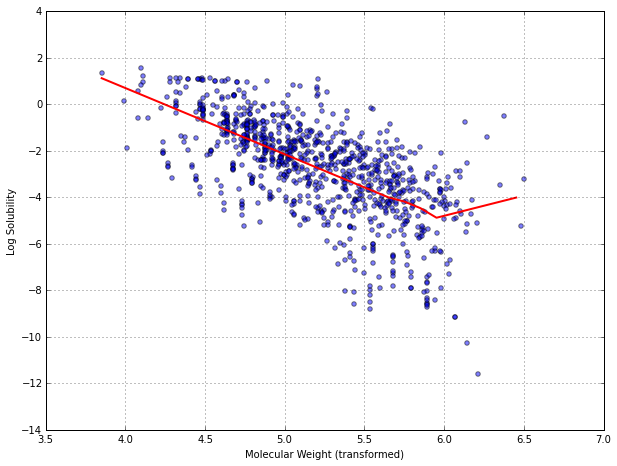There are several advantages to using MARS. First, the model automatically conducts feature selection; the model equation is independent of predictor variables that are not involved with any of the final model features. The second advantage is interpretability. Each hinge feature is responsible for modeling a specific region in the predictor space using a piecewise linear model. When the MARS model is additive, the contribution of each predictor can be isolated without the need to consider the others. This can be used to provide clear interpretation of how each predictor relates to the outcome. Finally, the MARS model requires very little pre-processing of the data; data transformation and the filtering of predictors are not needed. Correlated predictors do not drastically affect model performance, but they can complicate model interpretation.

Another method to help understand the nature of how the predictors affect the model is to quantify their importance to the model. For MARS, one technique for doing this is to track the reduction in the root mean squared error (as measured using the GCV statistic) that occurs when adding a particular feature to the model. This reduction is attributed to the original predictors associated with the feature. These improvements in the model can be aggregated for each predictor as a relative measure of the impact on the model.

## 7.3 Support Vector Machines¶

SVMs are a class of powerful, highly flexible modeling techniques. The theory behind SVMs was originally developed in the context of classification models. For regression, it is motivated in the framework of robust regression where we seek to minimize the effect of outliers on the regression equations. Also, there are several flavors of support vector regression and we focus on one particular technique called $\epsilon$-insentitive regression.

Recall that linear regression seeks to find parameter estimates that minimize SSE. One drawback of minimizing SSE is that the parameter estimates can be influenced by just one observation that falls far from the overall trend in the data. When data may contain influential observations, an alternative minimization metric that is less sensitive, such as the Huber function, can be used to find the best parameter estimates. This function uses the squared residuals when they are "small" and uses the absolute residuals when the residuals are large.

SVMs for regression use a function similar to the Huber function, with an important difference. Given a threshold set by the user (denoted as $\epsilon$), data points with residuals within the threshold do not contribute to the regression fit while data points with an absolute difference greater than the threshold contribute a linear-scale amount. There are several consequences to this approach. First, since the squared residuals are not used, large outliers have a limited effect on the regression equation. Second, samples that the model fits well (i.e., the residuals are small) have no effect on the regression equation. In fact, if the threshold is set to a relatively large value, then the outliers are the only points that define the regression line. This is somewhat counterintuitive: the poorly predicted points define the line. However, this approach has been shown to be very effective in defining the mode.

The SVM regression coefficients minimize $$Cost \times \sum_{i=1}^n L_{\epsilon} (y_i - \hat{y}_i) + \sum_{j=0}^P \beta_j^2,$$ where $L_{\epsilon} (\cdot)$ is the $\epsilon$-insensitive function. The $Cost$ parameter is the cost penalty that is set by the user, which penalizes large residuals. The penalty here is written as the reverse of ridge regression or weight decay in neural networks since it is attached to residuals and not the parameters.

Recall that the simple linear regression model predicted new samples using linear combinations of the data and parameters. For a new sample, $u$, the prediction equation is $$\hat{y} = \beta_0 + \sum_{j=1}^P \beta_j u_j$$ The linear support vector machine prediction function is very similar. The parameter estimates can be written as functions of a set of unknown parameters $(\alpha_i)$ and the training set data points so that $$\hat{y} = \beta_0 \sum_{j=1}^P \beta_j u_j = \beta_0 + \sum_{j=1}^P \sum_{i = 1}^n \alpha_i x_{ij} u_j = \beta_0 + \sum_{i = 1}^n \alpha_i (\sum_{j=1}^P x_{ij} u_j).$$

There are several aspects of this equation worth pointing out. First, there are as many $\alpha$ parameters as there are data points. From the standpoint of classical regression modeling, this model would be considered $over-parameterized$; typically, it is better to estimate fewer parameters than data points. However, the use of the cost value effectively regularizes the model to help alleviate this problem.

Second, the individual training set data points (i.e., the $x_{ij}$) are required for new predictors. When the training set is large, this makes the prediction equation less compact than other techniques. However, for some percentage of the training set samples, the $\alpha_i$ parameters will be exactly zero, indicating that they have no impact on the prediction equation. The data points associated with an $\alpha_i$ parameter of zero are the training set samples that are within $\pm \epsilon$ of the regression line. As a consequence, only a subset of the training set data points, where $\alpha \neq 0$, are needed for prediction. Since the regression line is determined using these samples, they are called the support vectors as they support the regression line.

In :
np.random.seed(3)

# toy example
x_sim = np.random.uniform(-2.5, 2.5, 100)
y_sim = 1 + 4*x_sim + np.random.normal(0, 1, 100)

# arbitrarily set outlier
xmin_idx = np.argmin(x_sim)
y_sim[xmin_idx] = 10

In :
# simple linear regression
from sklearn.linear_model import LinearRegression

ols = LinearRegression()
ols.fit(x_sim[:, np.newaxis], y_sim)
ols_pred = ols.predict(x_sim[:, np.newaxis])

print "y = {0} + {1} x".format(ols.intercept_, ols.coef_)

y = 1.11602295026 + 3.6516500913 x

In :
# support vectors machine regression
from sklearn.svm import SVR

eps = 0.1

svr = SVR('linear', epsilon = eps)
svr.fit(x_sim[:, np.newaxis], y_sim)
svr_pred = svr.predict(x_sim[:, np.newaxis])

print "y = {0} + {1} x".format(svr.intercept_, -svr.coef_)

y = 0.981450075744 + 3.87493669291 x

In :
plt.scatter(x_sim, y_sim, alpha=0.5, s=26)
plt_ols, = plt.plot(x_sim, ols_pred, 'g')
plt_svr, = plt.plot(x_sim, svr_pred, color='r')

plt.xlabel("Predictor")
plt.ylabel("Outcome")
plt.ylim(-11, 11)
plt.legend([plt_ols, plt_svr], ['Least Squares', 'SVM'], loc = 4)

Out:
<matplotlib.legend.Legend at 0x112789e50>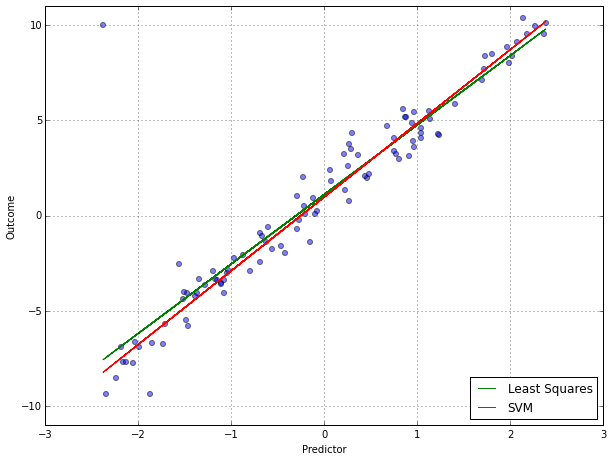In :
svr_residuals = np.delete(svr_pred - y_sim, xmin_idx, 0)

plt.scatter(np.delete(x_sim, xmin_idx, 0), svr_residuals, alpha=0.5, s=26)
plt.xlim(-3, 3)
plt.plot(plt.xlim(), (eps, eps), 'g--', linewidth=2)
plt.plot(plt.xlim(), (-eps, -eps), 'g--', linewidth=2)
plt.xlabel('Predicted Value')
plt.ylabel('Residual')

Out:
<matplotlib.text.Text at 0x112791bd0>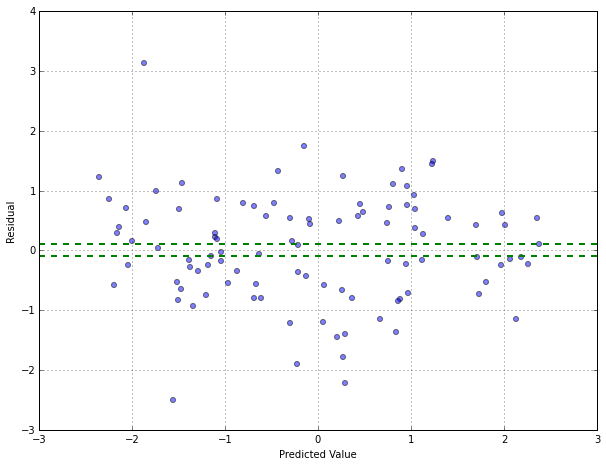In :
print "Out of 100 data points, {0} of these were support vectors.".format(np.sum(np.abs(svr_residuals) >= eps))

Out of 100 data points, 94 of these were support vectors.


Note that in the previous equation, the new samples enter into the prediction function as sum of cross products with the new sample values. In matrix algebra terms, this corresponds to a dot product (i.e., $\pmb{x}^T \pmb{u}$). The regression equation can be rewritten more generally as $$f(\pmb{u}) = \beta_0 + \sum_{i=1}^n \alpha_i K(\pmb{x}_i, \pmb{u}),$$ where $K (\cdot)$ is called the kernel function. When predictors enter the model linearly, the kernel function reduces to a simple sum of cross products shown above: $$K(\pmb{x}_i, \pmb{u}) = \sum_{j=1}^P x_{ij}u_j = \pmb{x}_i^T \pmb{u}$$. However, there are other types of kernel functions that can be used to generalize the regression model and encompass nonlinear functions of the predictors: $$\text{polynomial} = (\phi(\pmb{x}^T \pmb{u}) + 1)^{degree}$$ $$\text{radial basis function} = \exp(- \sigma\| \pmb{x}^T - \pmb{u} \|^2)$$ $$\text{hyperbolic tangent} = \tanh(\phi(\pmb{x}^T \pmb{u}) + 1),$$ where $\phi$ and $\sigma$ are scaling parameters. Since these functions of the predictors lead to nonlinear models, this generalization is often called the "kernel trick".

In :
np.random.seed(3)

# sin wave
x_sim = np.random.uniform(2, 10, 145)
y_sim = np.sin(x_sim) + np.random.normal(0, 0.4, 145)

# arbitrarily set outlier
x_outliers = np.arange(2.5, 5, 0.5)
y_outliers = -5*np.ones(5)

x_sim_idx = np.argsort(np.concatenate([x_sim, x_outliers]))
x_sim = np.concatenate([x_sim, x_outliers])[x_sim_idx]
y_sim = np.concatenate([y_sim, y_outliers])[x_sim_idx]

In :
# simple linear regression
from sklearn.linear_model import LinearRegression

ols = LinearRegression()
ols.fit(np.sin(x_sim[:, np.newaxis]), y_sim)
ols_pred = ols.predict(np.sin(x_sim[:, np.newaxis]))

print "y = {0} + {1} sin(x)".format(ols.intercept_, ols.coef_)

y = -0.145817806709 + 1.10984549112 sin(x)

In :
# support vectors machine regression
from sklearn.svm import SVR

eps = 0.1

svr = SVR('rbf', epsilon = eps)
svr.fit(x_sim[:, np.newaxis], y_sim)
svr_pred = svr.predict(x_sim[:, np.newaxis])

In :
plt.scatter(x_sim, y_sim, alpha=0.5, s=26)
plt_ols, = plt.plot(x_sim, ols_pred, 'g')
plt_svr, = plt.plot(x_sim, svr_pred, color='r')

plt.xlabel("Predictor")
plt.ylabel("Outcome")
plt.ylim(-5.2, 2.2)
plt.legend([plt_ols, plt_svr], ['Least Squares', 'SVM'], loc = 4)

Out:
<matplotlib.legend.Legend at 0x112a2cdd0>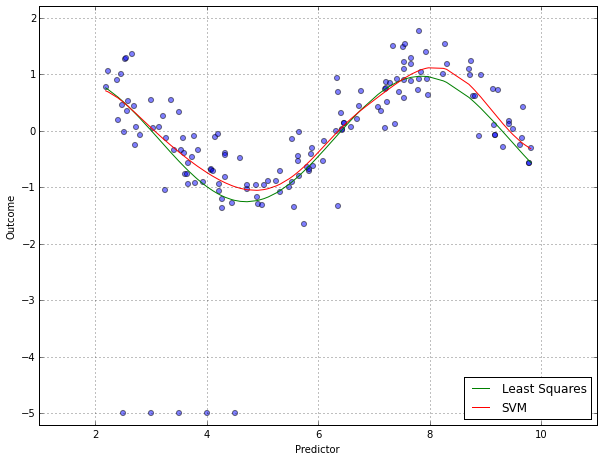A linear regression model with a term for $sin(x)$ was fit to the data and the regression line is pulled towards the outlying points. An SVM model with a radial basis kernel function is represented by the red line and it better describes the overall structure of the data.

The kernel function should be used depending on the problem. The radial basis function has been shown to be very effective. However, when the regression line is truly linear, the linear kernel function will be a better choice.

Note that some of the kernel functions have extra parameters. These parameters, along with the cost value, constitute the tuning parameters for the model. In case of the radial basis function, it is suggested to estimate the distribution of $\| x - x^, \|$ from the training set points and use the midpoint of the 10th and 90th percentiles for $\sigma$, instead of searching over a grid of candidate values.

The cost function is the main tool for adjusting the complexity of the model. When the cost is large, the model becomes very flexible since the effect of error is amplified. When the cost is small, the model will "stiffen" and become less likely to over-fit (but more likely to under-fit) because the contribution of the squared parameters is proportionally large in the modified error function. One could also tune the model over the size of the funnel (e.g., $\epsilon$). However, there is a relationship between $\epsilon$ and the cost parameter. Since the cost provides more flexibility for tuning the model, we suggest fixing a value for $\epsilon$ and tuning over the other kernel parameters.

Since the predictors enter into the model as the sum of cross products, differences in the predictor scales can affect the model. Therefore, we recommend centering and scaling the predictors prior to building an SVM model.

SVMs were applied to the solubility data.

In :
from pprint import pprint

opt_sigma = 0.0039

svr = SVR(kernel='rbf', gamma=opt_sigma, epsilon=0.1)
svr_params = {
'C': np.logspace(-2, 11, num=14, base=2),
}
pprint(svr_params)

{'C': array([  2.50000000e-01,   5.00000000e-01,   1.00000000e+00,
2.00000000e+00,   4.00000000e+00,   8.00000000e+00,
1.60000000e+01,   3.20000000e+01,   6.40000000e+01,
1.28000000e+02,   2.56000000e+02,   5.12000000e+02,
1.02400000e+03,   2.04800000e+03])}

In :
from sklearn.grid_search import GridSearchCV
from sklearn.cross_validation import ShuffleSplit

cv = ShuffleSplit(trainX.shape, n_iter=10, random_state=3)

gs_svr = GridSearchCV(svr, svr_params, cv=cv, scoring="mean_squared_error", n_jobs=-1)
gs_svr.fit(trainX.values, trainY.values[:,0])

Out:
GridSearchCV(cv=ShuffleSplit(951, n_iter=10, test_size=0.1, random_state=3),
estimator=SVR(C=1.0, cache_size=200, coef0=0.0, degree=3, epsilon=0.1, gamma=0.0039,
kernel='rbf', max_iter=-1, probability=False, random_state=None,
shrinking=True, tol=0.001, verbose=False),
fit_params={}, iid=True, loss_func=None, n_jobs=-1,
param_grid={'C': array([  2.50000e-01,   5.00000e-01,   1.00000e+00,   2.00000e+00,
4.00000e+00,   8.00000e+00,   1.60000e+01,   3.20000e+01,
6.40000e+01,   1.28000e+02,   2.56000e+02,   5.12000e+02,
1.02400e+03,   2.04800e+03])},
pre_dispatch='2*n_jobs', refit=True, score_func=None,
scoring='mean_squared_error', verbose=0)
In :
gs_grid_rmse = [np.sqrt(-d) for d in gs_svr.grid_scores_]

plt.plot(np.logspace(-2, 11, num=14, base=2), gs_grid_rmse, '-x')
plt.xscale('log', basex=2)
plt.xlim(2**-2.5, 2**11.5)
plt.xlabel('Cost')
plt.ylabel('RMSE (Cross-Validation)')

Out:
<matplotlib.text.Text at 0x112a55090>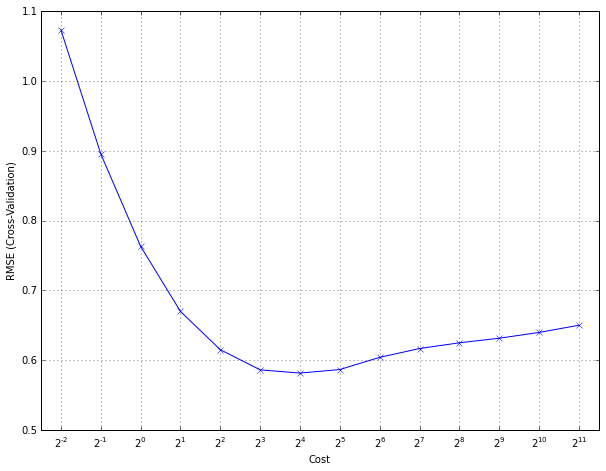In :
print "Best cost value associated with the smallest RMSE was {0}".format(gs_svr.best_params_)

Best cost value associated with the smallest RMSE was {'C': 16.0}


SVMs with polynomial kernel was also evaluated. We tuned over the cost, the polynomial degree, and a scale factor.

In :
# polynomial kernel
svr_poly = SVR(kernel='poly', epsilon=0.1)

svr_poly_params = {
'C': np.logspace(-2, 5, num=8, base=2),
'gamma': [0.001, 0.005, 0.01],
'degree': [1, 2]
}
pprint(svr_poly_params)

{'C': array([  0.25,   0.5 ,   1.  ,   2.  ,   4.  ,   8.  ,  16.  ,  32.  ]),
'degree': [1, 2],
'gamma': [0.001, 0.005, 0.01]}

In :
cv = ShuffleSplit(trainX.shape, n_iter=10, random_state=3)

gs_svr_poly = GridSearchCV(svr_poly, svr_poly_params, cv=cv, scoring="mean_squared_error", n_jobs=-1)
gs_svr_poly.fit(trainX.values, trainY.values[:,0])

Out:
GridSearchCV(cv=ShuffleSplit(951, n_iter=10, test_size=0.1, random_state=3),
estimator=SVR(C=1.0, cache_size=200, coef0=0.0, degree=3, epsilon=0.1, gamma=0.0,
kernel='poly', max_iter=-1, probability=False, random_state=None,
shrinking=True, tol=0.001, verbose=False),
fit_params={}, iid=True, loss_func=None, n_jobs=-1,
param_grid={'C': array([  0.25,   0.5 ,   1.  ,   2.  ,   4.  ,   8.  ,  16.  ,  32.  ]), 'degree': [1, 2], 'gamma': [0.001, 0.005, 0.01]},
pre_dispatch='2*n_jobs', refit=True, score_func=None,
scoring='mean_squared_error', verbose=0)
In :
def split_rmse_scores(grid_scores, scale, degree):
'''get the grid scores for each combination of scale and degree'''
rmse_scores = [np.sqrt(-d) for d in grid_scores if (d['degree'] == degree and d['gamma'] == scale)]
return rmse_scores

In :
fig, (ax1, ax2, ax3) = plt.subplots(1, 3, sharey=True)

line1, = ax1.plot(np.logspace(-2, 5, num=8, base=2), split_rmse_scores(gs_svr_poly.grid_scores_, 0.001, 1), '-x')
line2, = ax1.plot(np.logspace(-2, 5, num=8, base=2), split_rmse_scores(gs_svr_poly.grid_scores_, 0.001, 2), '-o')
ax1.set_xscale('log', basex=2)
ax1.set_xlim(2**-2.5, 2**5.5)
ax1.set_title('scale: 0.001')

ax2.plot(np.logspace(-2, 5, num=8, base=2), split_rmse_scores(gs_svr_poly.grid_scores_, 0.005, 1), '-x')
ax2.plot(np.logspace(-2, 5, num=8, base=2), split_rmse_scores(gs_svr_poly.grid_scores_, 0.005, 2), '-o')
ax2.set_xscale('log', basex=2)
ax2.set_xlim(2**-2.5, 2**5.5)
ax2.set_title('scale: 0.005')

ax3.plot(np.logspace(-2, 5, num=8, base=2), split_rmse_scores(gs_svr_poly.grid_scores_, 0.01, 1), '-x')
ax3.plot(np.logspace(-2, 5, num=8, base=2), split_rmse_scores(gs_svr_poly.grid_scores_, 0.01, 2), '-o')
ax3.set_xscale('log', basex=2)
ax3.set_xlim(2**-2.5, 2**5.5)
ax3.set_title('scale: 0.01')

fig.legend([line1, line2], ('Degree 1', 'Degree 2'), loc='upper center', ncol=2, frameon=False)
fig.text(0.08, 0.5, 'RMSE (Cross-Validation)', ha='center', va='center', rotation=90)
fig.text(0.5, 0.07, 'Cost', ha='center', va='center')

Out:
<matplotlib.text.Text at 0x112f92110>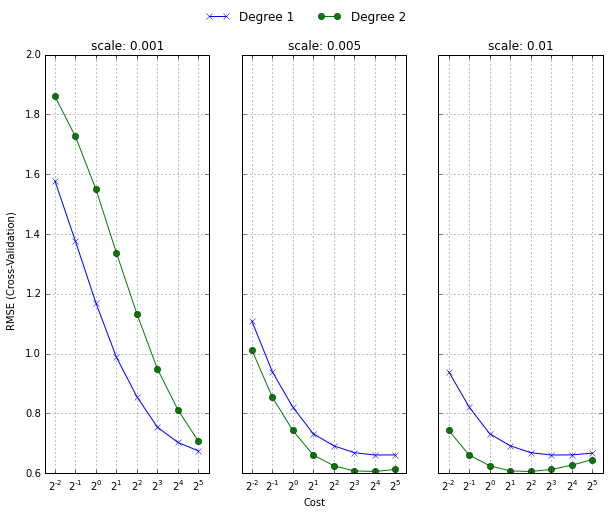In general, quadratic models have smaller error rates than the linear models. Also, models associated with large-scale factors have better performance. It is important to point out that tuning the radial basis function kernel parameters was easier than tuning the polynomial model (which has more parameters).

## 7.4 K-Nearest Neighbors¶

The KNN approach simply predicts a new sample using the K-cloest samples from the training set. It cannot be clearly summarized by a model. Instead, its construction is solely based on the individual samples from the training data. To predict a new sample for regression, KNN identifies that sample's KNNs in the predictor space. The predicted response for the new sample is then the mean of the K neighbors' responses. Other summary statistics, such as the median, can also be used in place of the mean to predict the new sample.

The basic KNN method depends on how the user defines distance between samples. Euclidean distance is the most commonly used metric and is defined as follows: $$(\sum_{j=1}^P (x_{aj} - x_{bj})^2)^{1/2},$$ where $\pmb{x}_a$ and $\pmb{x}_b$ are two individual samples. Minkowski distance is a generalization of Euclidean distance and is defined as $$(\sum_{j=1}^P |x_{aj} - x_{bj}|^q)^{1/q},$$ where $q > 0$. It is easy to see that when $q = 2$, then Minkowski distance is the same as Euclidean distance. When $q = 1$, then Minkowski distance is equivalent to Manhattan distance, which is a common metric used for samples with binary predictors.

Because the KNN method fundamentally depends on distance between samples, the scale of the predictors can have a dramatic influence on the distances among samples. That is, predictors with the largest scales will contribute most to the distance between samples. To avoid the potential bias and to enable each predictor to contribute equally to the distance calculation, we recommend that all predictors be centered and scaled prior to performing KNN.

In addition to the issue of scaling, using distances between samples can be problematic if one or more of the predictor values for a sample is missing, since it is then not possible to compute the distance between samples. If this is the case, then the analyst has a couple of options. First, either the samples or the predictors can be excluded from the analysis. If a predictor contains a sufficient amount of information across the samples, then an alternative approach is to impute the missing data using a naive estimator such as the mean of the predictor, or a nearest neighbor approach that uses only the predictors with complete information.

Upon pre-processing the data and selecting the distance metric, the next step is to find the optimal number of neighbors. Like tuning parameters from other models, K can be determined by resampling.

In :
from sklearn.neighbors import KNeighborsRegressor

knnreg = KNeighborsRegressor()

knn_params = {
'n_neighbors': np.arange(1, 21, 1)
}
pprint(knn_params)

cv = ShuffleSplit(trainX.shape, n_iter=10, random_state=3)

gs_knnreg = GridSearchCV(knnreg, knn_params, cv=cv, scoring='mean_squared_error', n_jobs=-1)
gs_knnreg.fit(trainX.values, trainY.values)

{'n_neighbors': array([ 1,  2,  3,  4,  5,  6,  7,  8,  9, 10, 11, 12, 13, 14, 15, 16, 17,
18, 19, 20])}

Out:
GridSearchCV(cv=ShuffleSplit(951, n_iter=10, test_size=0.1, random_state=3),
estimator=KNeighborsRegressor(algorithm='auto', leaf_size=30, metric='minkowski',
metric_params=None, n_neighbors=5, p=2, weights='uniform'),
fit_params={}, iid=True, loss_func=None, n_jobs=-1,
param_grid={'n_neighbors': array([ 1,  2,  3,  4,  5,  6,  7,  8,  9, 10, 11, 12, 13, 14, 15, 16, 17,
18, 19, 20])},
pre_dispatch='2*n_jobs', refit=True, score_func=None,
scoring='mean_squared_error', verbose=0)
In :
gs_knnreg_rmse = [np.sqrt(-d) for d in gs_knnreg.grid_scores_]

plt.plot(np.arange(1, 21, 1), gs_knnreg_rmse, '-x')
plt.xlim(None, 21)
plt.xlabel('#Neighbors')
plt.ylabel('RMSE (Cross-Validation)')

Out:
<matplotlib.text.Text at 0x112fc6d90>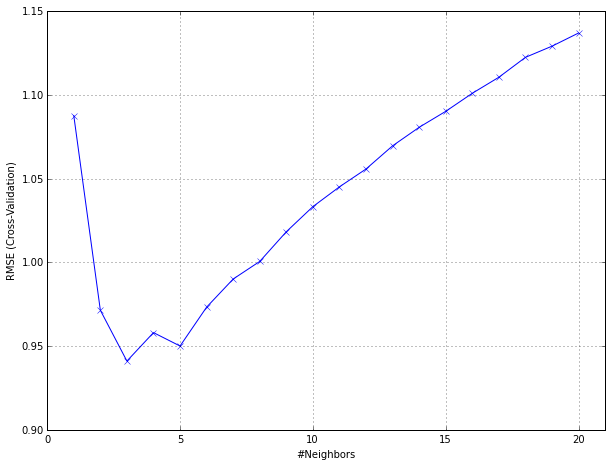Typically, small values of K usually over-fit and large values of K ususally underfit the data.

The elementray version of KNN is intuitive and straightforward and can produce decent predictions, especially when the response is dependent on the local predictor structure. However, this version does have some notable problems, of which researchers have sought solutions. Two commonly noted problems are computational time and the disconnect between local structure and the predictive ability of KNN.

First, to predict a sample, distances between the sample and all other samples must be computed. Computational time therefore increases with n because the training data must be loaded into memory and because distances between the new sample and all of the training samples must be computed. To mitigate this problem, one can replace the original data with a less memory-intensive representation of the data that describes the locations of the original data. One specific example of this representation is a k-dimensional tree. A k-d tree orthogonally partitions the predictor space using a tree approach but with different rules. After the tree has been grown, a new sample is placed through the structure. Distances are only computed for those training observations in the tree that are close to the new sample. This approach provides significant computational improvements, especially when the number of training samples is much larger than the number of predictors.

The KNN method can have poor predictive performance when local predictor structure is not relevent to the response. Irrelevant or noisy predictors are one culprit, since these can cause similar samples to be driven away from each other in the predictor space. Hence, removing irrelevant, noise-laden predictors is a key pre-processing step for KNN. Another approach to enhancing KNN predictivity is to weight the neighbors' contribution to the prediction of a new sample based on their distances to the new sample. In this variation, training samples that are closer to the new sample contribute more to the predicted response, while those that are farther away contribute less to the predicted response.